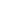Flat 50% off

Ends in

• JEE Main
•• Mathematics
• Back to Doubt Clearing

## If $f\left(\frac{x}{y}\right)=\frac{f(x)}{f(y)} \quad \forall x, y \in R$ and $f^{\prime}(1)$ exists, and area under the curve $f(x)$ bounded by x-axis $x=0$ and $x = 1$ is $\frac {1}{3}$, then find $\lim _{n \rightarrow \infty} \sum_{r=1}^{n} e^{r / n} f\left(\frac{\sqrt{r}}{n}\right)$.

### Asked By priya

Updated Fri, 07 Jun 2019 03:54 pm